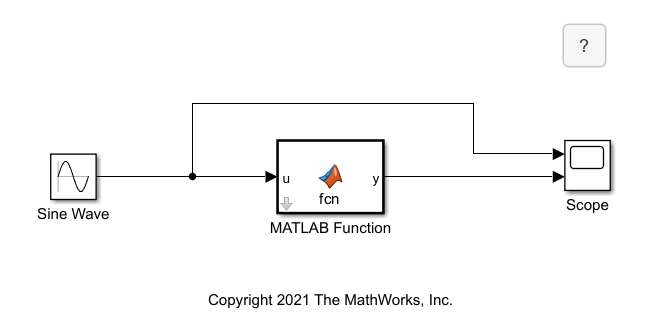## Configure MATLAB Function Block Parameter Variables

You can use parameter variables in MATLAB Function blocks to use variables defined in the MATLAB® workspace, model workspace, a masked subsystem workspace, or a Simulink® data dictionary as input arguments. Simulink searches for and selects variables used by parameter variables by using the same rules for symbol resolution. See Symbol Resolution. If a variable of the same name exists in more than one of the workspaces visible to the block, the block uses the variable closest to the block in the workspace hierarchy. If the MATLAB Function block is in a masked subsystem, the block uses the variable in the subsystem workspace. Otherwise, the block uses the variable in the model workspace, followed by using the variable in the MATLAB base workspace and in an associated Simulink data dictionary, in that order.

To define parameter variables, use the Symbols pane, Property Inspector, or the Model Explorer. Set the Scope property of the selected variable to `Parameter`. Parameter variables do not create block ports. For more information about defining variables in MATLAB Function blocks, see Create and Define MATLAB Function Block Variables.

### Control Parameter Variables with Masks

You can directly assign values to parameter variables in a MATLAB Function block by adding a mask to the block, creating a parameter in the mask, and giving the parameter the same name as the parameter variable. For more information about creating masks for blocks, see Masking Fundamentals. After you mask the block, you can adjust the parameter variable properties by using the Property Inspector.

This example uses a masked MATLAB Function block with a parameter variable.The MATLAB function multiplies the parameter variable `const` by the value of the Sine Wave block signal. Click the arrow icon on the MATLAB Function block to view the code.

```function y = fcn(u, const) ```
```y = const*u; ```

You can adjust the value of `const` by double-clicking the MATLAB Function block. The block mask defines the Set sine wave multiplier parameter, which you can edit in the Mask Editor. The MATLAB Function block defines `const` as data with Scope set to `Parameter` in the Property Inspector. You can see this property in the Property Inspector when you select `const` in the Symbols pane.The parameter variable and the mask parameter must have the same name. In this example, changing the parameter variable name without changing the mask parameter name causes an error. If you are using a non-tunable parameter, ensure that the Tunable property is cleared in the Property Inspector.

### Control Parameter Variables in Workspaces

If you want multiple MATLAB Function blocks to access the same variable in a model, you can define the variable in the model workspace and add parameter variables to each MATLAB function that points to that variable. You can define the variable directly in the model workspace, load the variable in a MAT-file or MATLAB file, or create the variable with custom MATLAB code. For more information, see Specify Source for Data in Model Workspace.

If you want to use the variable in MATLAB Function blocks in multiple models, you can define the variable in the MATLAB base workspace by defining the variable in the Command Window or by loading a MAT-file. MATLAB Function blocks access variables defined in the MATLAB workspace until you close MATLAB.

You can also define variables using a Simulink data dictionary. For more information, see What Is a Data Dictionary?.